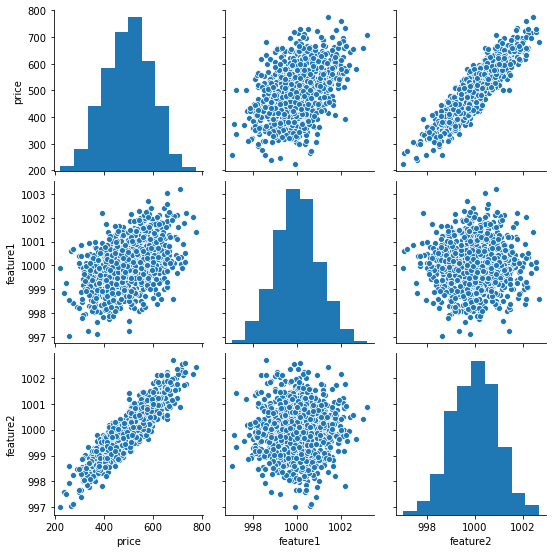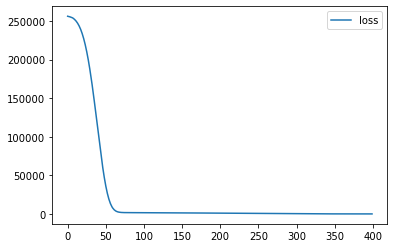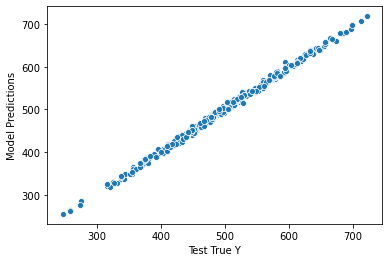Below is a small example showing how to utilize Keras/Tensorflow 2.0 to predict a value utilizing a small dataset. More explanations to follow in the Jupyter notebook below…

## Keras / Tensorflow Basics – A Simple Example

The dataset utilized here is fake, for the sake of example use only. It contains a price and two “features”. We’re assuming the dataset is a price listing of gemstones, and based on the features we can predict what the price of a new gemstone added to the list may be.

The data can be found here.

In :
```#Imports
import pandas as pd
import numpy as np
import seaborn as sns
```

### Data

In :
```df = pd.read_csv('Keras/fake_reg.csv')
```
In :
```df.head()
```
Out:
price feature1 feature2
0 461.527929 999.787558 999.766096
1 548.130011 998.861615 1001.042403
2 410.297162 1000.070267 998.844015
3 540.382220 999.952251 1000.440940
4 546.024553 1000.446011 1000.338531
In :
```sns.pairplot(df)
```
Out:
`<seaborn.axisgrid.PairGrid at 0x18188b92e48>`This is a very simply dataset, but the pairplot can show us how the two features may correlate to pricing.

### Training the Model

In :
```from sklearn.model_selection import train_test_split
```
In :
```#We need .values because it's best to pass in numpy arrays due to how tensorflow works
X = df[['feature1', 'feature2']].values
y = df['price'].values
```
In :
```#Split into test/train
X_train, X_test, y_train, y_test = train_test_split(X, y, test_size=0.3, random_state=42)
```
In :
```#Scale data to be between 0 and 1
from sklearn.preprocessing import MinMaxScaler

scaler = MinMaxScaler()
```
In :
```#Calc params needed to scale later on
#Only perform on training set as to not influence based on test data
scaler.fit(X_train)
```
Out:
`MinMaxScaler()`
In :
```#Perform transformation
X_train = scaler.transform(X_train)
X_test = scaler.transform(X_test)
```

### Create Model – Neural Network

In :
```from tensorflow.keras.models import Sequential
from tensorflow.keras.layers import Dense, Activation
```

#### Choosing an optimizer and loss

Keep in mind what kind of problem you are trying to solve:

``````# For a multi-class classification problem
model.compile(optimizer='rmsprop',
loss='categorical_crossentropy',
metrics=['accuracy'])

# For a binary classification problem
model.compile(optimizer='rmsprop',
loss='binary_crossentropy',
metrics=['accuracy'])

# For a mean squared error regression problem
model.compile(optimizer='rmsprop',
loss='mse')``````
In :
```#Add multiple layers into sequential with the number of neurons needed
model = Sequential()

```
In :
```#Fit
model.fit(x=X_train,y=y_train,epochs=400)
```
In :
```#Grab losses and stick into a dataframe
loss_df = pd.DataFrame(model.history.history)
```
In :
```#Plot out of the losses
#I.E. show how the model continually improves until no more improvement is possible
loss_df.plot()
```
Out:
`<AxesSubplot:>`### Model Evaluation

There are several ways to evaluate how this model performs

#### Evaluate MSE

In :
```#Evaluate MSE for test vs training set
training_score = model.evaluate(X_train,y_train,verbose=0)
test_score = model.evaluate(X_test,y_test,verbose=0)
```
In :
```training_score
```
Out:
`26.82504653930664`
In :
```test_score
```
Out:
`28.019241333007812`

#### Run Predictions against test set

In :
```test_predictions = model.predict(X_test)
```
In :
```#Put in a series
test_predictions = pd.Series(test_predictions.reshape(300,))
```
In :
```#Concatenate into one dataframe
pred_df = pd.DataFrame(y_test,columns=['Test True Y'])
pred_df = pd.concat([pred_df,test_predictions], axis=1)

pred_df.columns = ['Test True Y', 'Model Predictions']
```
In :
```#Compare Correlation of Test/Train
sns.scatterplot(x='Test True Y', y='Model Predictions', data=pred_df)
```
Out:
`<AxesSubplot:xlabel='Test True Y', ylabel='Model Predictions'>`#### Revisit MSE along with Other Metrics

In :
```from sklearn.metrics import mean_absolute_error, mean_squared_error
```
In :
```#MAS
#I.E. On average, how many dollars off are we?
mean_absolute_error(pred_df['Test True Y'], pred_df['Model Predictions'])
```
Out:
`4.309533628692207`
In :
```#MSE
mean_squared_error(pred_df['Test True Y'], pred_df['Model Predictions'])
```
Out:
`28.019247775153865`
In :
```#RMSE
mean_squared_error(pred_df['Test True Y'], pred_df['Model Predictions'])**0.5
```
Out:
`5.293321053474261`

### Run predictions on new data

Let’s pretend we want to add a gem, without a price, to the model and predict a price.

We’ll create a new gem with two features

In :
```new_gem = [[998,1000]]
```
In :
```#Remember to scale the data
new_gem = scaler.transform(new_gem)
```
In :
```model.predict(new_gem)
```
Out:
`array([[422.68384]], dtype=float32)`

So, here we’re predicting the price of the new gem will be approx. \$423 dollars with those two features listed.

### Save the model for future use

In :
```from tensorflow.keras.models import load_model
```
In :
```model.save('my_gem_model.h5')
```
In :
```later_model = load_model('my_gem_model.h5')
```

WordPress conversion from Keras Basics.ipynb by nb2wp v0.3.1